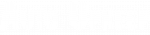# Engine Size

Q: What does the L mean in the description of an engine? For example, my car has a 2.4-L engine?

A: The 2.4-L stands for the displacement of the engine in liters. This is also considered the engine size. Displacement is the combined volume of air in the engine’s cylinders – from the pistons’ bottommost point (bottom dead center) to topmost point (top dead center). This distance the piston travels is defined as the stroke. To calculate the displacement, the diameter of the cylinder (i.e., bore) and the stroke are needed. The basic mathematical formula for calculating the volume of a cylinder is: volume = (pi) (r2) (h). Since the bore is the diameter and not the radius (r), divide the bore by 2 to get “r”. The “h” is the stroke and “pi” is the constant 3.14. To obtain the units in liters, the “r” and “h” need to be in centimeters. Then multiply the volume of one cylinder by the total number of cylinders and finally divide the total by 1000 to obtain liters (since 1000 cm3 = 1 L).